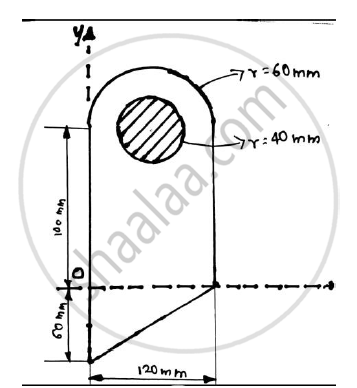# Determine the Position of the Centroid of the Plane Lamina. Shaded Portion is Removed. - Engineering Mechanics

Short Note

Determine the position of the centroid of the plane lamina. Shaded portion is removed.#### Solution

 FIGURE AREA(mm2) X co-ordinate Of centroid (mm) Y co-ordinate Of centroid (mm) Ax (mm2) Ay (mm2) Rectangle 120 x 100 =12000 120/2=60 120/2=60 720000 600000 Triangle 1/2120 x 60 =3600 120/3=40 -60/2=60 144000 -72000 Semicircle 1/2𝝅 x 602 =1800 𝝅=5654.8668 120/2=60 100+(4∗60)/(3x)=125.4648 339292.01 709486.68 Circle(Removed) - 𝝅 x 402=5026.5482 120/2=60 100 -301592.89 -502654.82 Total 16228.32 901699.12 734831.86

(Σ Ax)/(Σ A)=(901699.12)/(16228.32)= 55.56 mm
(Σ Ay)/(Σ A)=(734831.86)/(16228.32)= 45.28 mm
Centroid is at (55.56,45.28)mm

Concept: Centroid for Plane Laminas
Is there an error in this question or solution?

Share